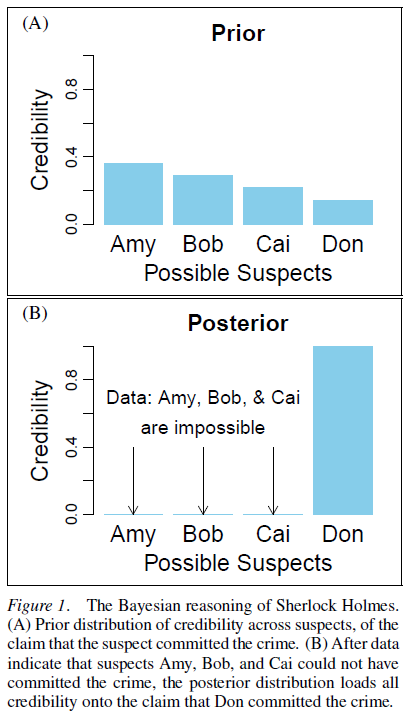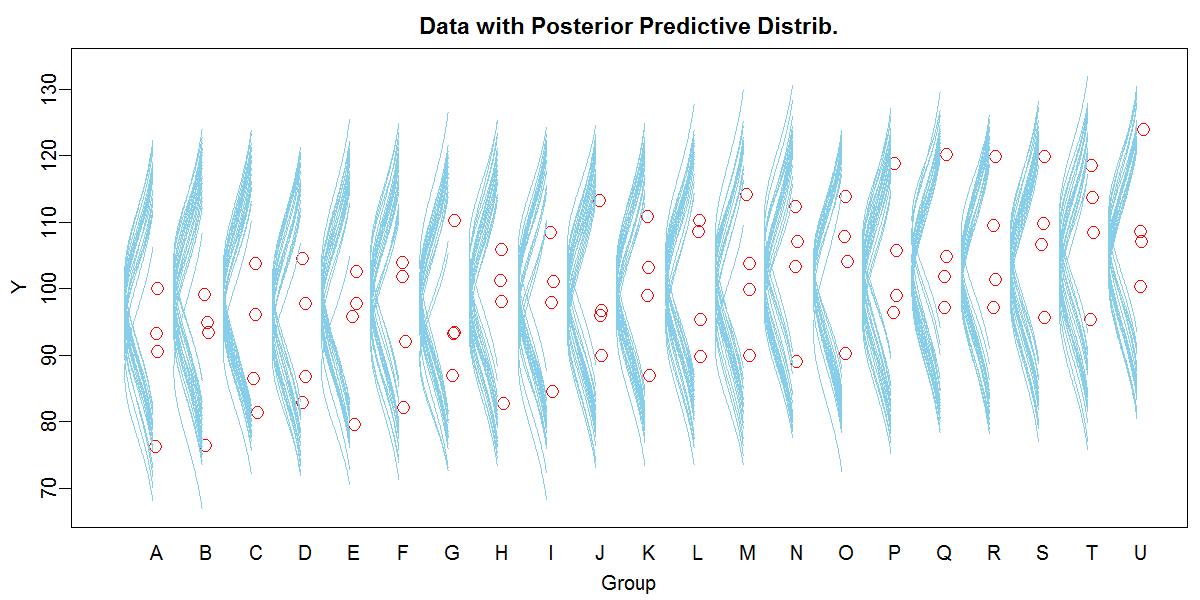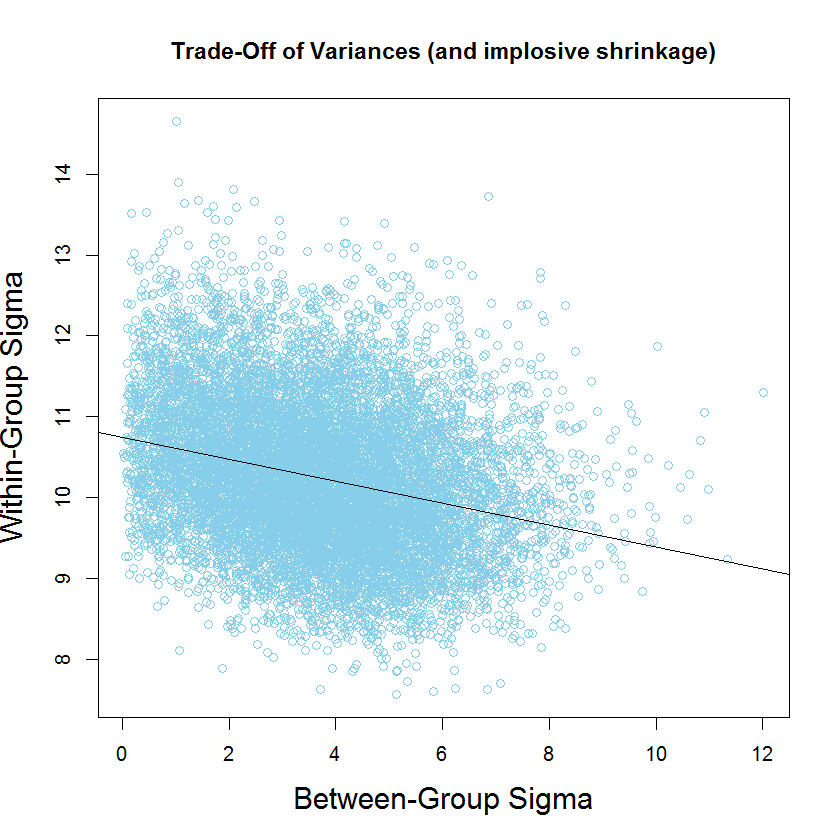## Sunday, April 16, 2017

### Bayesian workshops, June 2017

Upcoming multi-day workshops in doing Bayesian data analysis (2017):

## Wednesday, April 12, 2017

### New article: Bayesian for newcomersJust published: Bayesian data analysis for newcomers.

Abstract: This article explains the foundational concepts of Bayesian data analysis using virtually no mathematical notation. Bayesian ideas already match your intuitions from everyday reasoning and from traditional data analysis. Simple examples of Bayesian data analysis are presented, that illustrate how the information delivered by a Bayesian analysis can be directly interpreted. Bayesian approaches to null-value assessment are discussed. The article clarifies misconceptions about Bayesian methods that newcomers might have acquired elsewhere. We discuss prior distributions and explain how they are not a liability but an important asset. We discuss the relation of Bayesian data analysis to Bayesian models of mind, and we briefly discuss what methodological problems Bayesian data analysis is not meant to solve. After you have read this article, you should have a clear sense of how Bayesian data analysis works and the sort of information it delivers, and why that information is so intuitive and useful for drawing conclusions from data.

Accepted manuscript available at https://osf.io/preprints/psyarxiv/nqfr5.
View only:

## Saturday, April 8, 2017

### Trade-off of between-group and within-group variance (and implosive shrinkage)

Background: Consider data that would traditionally be analyzed as single-factor ANOVA; that is, a continuous metric predicted variable, $y$, and a nominal predictor, "Group." In particular, consider the data plotted as red dots here:A Bayesian approach easily allows a hierarchical model in which both the within-group and between-group variance are estimated. The hierarchical structure imposes shrinkage on the estimated group means. All of this explained in Chapter 19 of DBDA2E

The data above come from Exercise 19.1, which is designed to illustrate "implosive shrinkage." Because there are only a few data points in each group, and there are lots of groups with little variance from baseline, a reasonable description of the data merely collapses the group means close to baseline while expanding the estimate of within-group variance.

The purpose of the present post is to show the trade-off in the posterior distribution of the estimated within-group variance and between-group variance, while also providing another view of implosive shrinkage.

After running the data through the generic hierarchical model in Exercise 19.1, we can look at the posterior distribution of the within-group and between-group standard deviations. The code below produces the following plot:

mcmcMat = as.matrix( mcmcCoda )
openGraph()
plot( mcmcMat[,"aSigma"] , mcmcMat[,"ySigma"] ,
xlab="Between-Group Sigma" , ylab="Within-Group Sigma" ,
col="skyblue" , cex.lab=1.5 ,
main="Trade-Off of Variances (and implosive shrinkage)" )
abline( lm( mcmcMat[,"ySigma"] ~ mcmcMat[,"aSigma"] ) )Notice in the plot that as the between-group standard deviation gets larger, the within-group standard deviation gets smaller. Notice also the implosive shrinkage: The estimate of between-group standard deviation is "piled up" against zero.